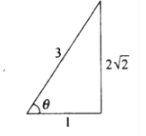Deepak Scored 45->99%ile with Bounce Back Crack Course. You can do it too!

# Find the value of the expression

Question:

Find the value of the expression sin (2 tan-1 1/3) + cos (tan-1 2√2).

Solution:

Given expression, sin (2 tan-1 1/3) + cos (tan-1 2√2)

$\sin \left(2 \tan ^{-1} \frac{1}{3}\right)=\sin \left(\sin ^{-1} \frac{2 \times \frac{1}{3}}{1+\left(\frac{1}{3}\right)^{2}}\right)\left(\because 2 \tan ^{-1} x=\sin ^{-1} \frac{2 x}{1+x^{2}}\right)$

$=\sin \left(\sin ^{-1} \frac{2 / 3}{10 / 9}\right)$

$=\sin \left(\sin ^{-1} \frac{3}{5}\right)=\frac{3}{5}$ $\left(\because \sin \left(\sin ^{-1} x\right)=x, x \in[-1,1]\right)$

$\cos \left(\tan ^{-1} 2 \sqrt{2}\right)=\cos \left(\cos ^{-1} \frac{1}{3}\right)=\frac{1}{3}$

$\left(\because \cos \left(\cos ^{-1} x\right)=x, x \in[-1,1]\right)$Hence,

$\sin \left(2 \tan ^{-1} \frac{1}{3}\right)+\cos \left(\tan ^{-1} 2 \sqrt{2}\right)=\frac{3}{5}+\frac{1}{3}=\frac{9+5}{15}=\frac{14}{15}$